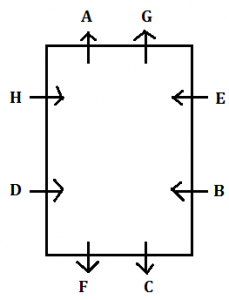Latest Banking jobs   »   Reasoning Ability Quiz For IBPS RRB...

# Reasoning Ability Quiz For IBPS RRB PO, Clerk Prelims 2021- 3rd August

Directions (1-5): Study the information carefully and answer the questions given below.
Eight persons are sitting around a rectangular table such that two persons sit on each side of the table. The ones sitting on the shorter side of the table face away from the center and the one sitting on the longer side of the table face toward the center. No two persons sitting opposite to each other are next to each other according to alphabetical series. E is one of the persons. A is immediate left to G, who sits at the shorter side of the table. H is 2nd right to F. C is immediate left to B, who sits opposite to D. A sits opposite to one of the immediate neighbor of C.

Q1. Who among the following sits opposite to H?
(a) E
(b) F
(c) C
(d) B
(e) none of these

Q2. Who among the following 4th to the left to the one who is 2nd left to A?
(a) E
(b) F
(c) C
(d) B
(e) none of these

Q3. Which of the following represents the position of G with respect to C?
(a) immediate left
(b) immediate right
(c) 2nd to the right
(d) 4trh to the left
(e) none of these

Q4. Which of the following statement is true regarding B?
(a) E faces B
(b) F sits 3rd left to B
(c) B and G sit on the same side of the table
(d) B faces towards the center of the table
(e) none of these

Q5. How many persons sit between D and H, when counted from the left to H?
(a) Two
(b) One
(c) Three
(d) Four
(e) None of these

Directions (6-10): Study the following arrangement carefully and answer the questions given below:
B 5 R 1 @ E K 4 F 7 © D A M 2 P 3 % 9 H I W 8 * 6 U J \$ V Q #

Q6. Which of the following is the fifth to the left of the seventeenth from the left end of the above arrangement?
(a) D
(b) W
(c) *
(d) 4
(e) None of these

Q7. Which of the following is exactly in the middle between D and U in the above arrangement?
(a) %
(b) H
(c) 9
(d) 3
(e) None of these

Q8. Four of the following five are alike in a certain way based on their position in the above arrangement and so form a group. Which is the one that does not belong to that group?
(a) R1E
(b) F7D
(c) M23
(d) 9HW
(e) UJ6

Q9. How many such symbols are there in the above arrangement each of which is immediately preceded by a number but not immediately followed by a consonant?
(a) None
(b) One
(c) Two
(d) Three
(e) More than three

Q10. How many such consonants are there in the above arrangement each of which is immediately followed by another consonant but not immediately preceded by a symbol?
(a) None
(b) One
(c) Two
(d) Three
(e) More than three

Directions (11-15): In these questions, a relationship between different elements is shown in the statements. The statements are followed by two conclusions. Give answer
Q11. Statement: A>B; G<D≤E; G≥F>B
Conclusion: I. G≥A II. B<E
(a) if only conclusion II is true.
(b) if only conclusion I is true.
(c) if neither conclusion I nor II is true.
(d) if either conclusion I or II is true.
(e) if both conclusions I and II are true.

Q12. Statement: A>T≥J; A≤S=H; I>T
Conclusion: I. H>J II. I>S
(a) if both conclusion I and II are true.
(b) if only conclusion I is true.
(c) if neither conclusion I nor II is true.
(d) if either conclusion I or II is true.
(e) if only conclusion II is true.

Q13. Statement: J≥K>L<M≥N; K≥O=T
Conclusion: I. J>O II. J=T
(a) if only conclusion II is true.
(b) if either conclusion I or II is true.
(c) if neither conclusion I nor II is true.
(d) if only conclusion I is true.
(e) if both conclusions I and II are true.

Q14. Statement: A> T=N; A>S>R; A<M
Conclusion: I.R<T II. N≤R
(a) if only conclusion II is true.
(b) if only conclusion I is true.
(c) if neither conclusion I nor II is true.
(d) if either conclusion I or II is true.
(e) if both conclusions I and II are true.

Q15. Statement: A<B≤C; F<M≤C; C>Q
Conclusion: I. Q≤F II. F>Q
(a) if only conclusion II is true.
(b) if either conclusion I or II is true.
(c) if neither conclusion I nor II is true.
(d) if only conclusion I is true.
(e) if both conclusions I and II are true.

Practice More Questions of Reasoning for Competitive Exams:

###### Target IBPS RRB PO and Clerk Prelims 2021

Solutions

Solutions (1-5):
Sol.S1.Ans(a)
S2.Ans(a)
S3.Ans(e)
S4.Ans(d)
S5.Ans(e)

S6. Ans.(a)
Sol. 5th to left of 17th from left= 17-5= 12th from left = D

S7. Ans.(c)
Sol. 9

S8. Ans.(e)
Sol. UJ6

S9. Ans.(d)
Sol.1@, 3%, 8*

S10. Ans.(a)
Sol. None

S11. Ans.(a)
Sol. I. G≥A(False) II. B<E(True)

S12. Ans.(b)
Sol. I. H>J(True) II. I>S (False)

S13. Ans.(b)
Sol. I. J>O(False) II. J=T (False)

S14. Ans.(d)
Sol. I.R<T(False) II. N≤R(False)

S15. Ans.(c)
Sol. I. Q≤F (False) II. F>Q(False)